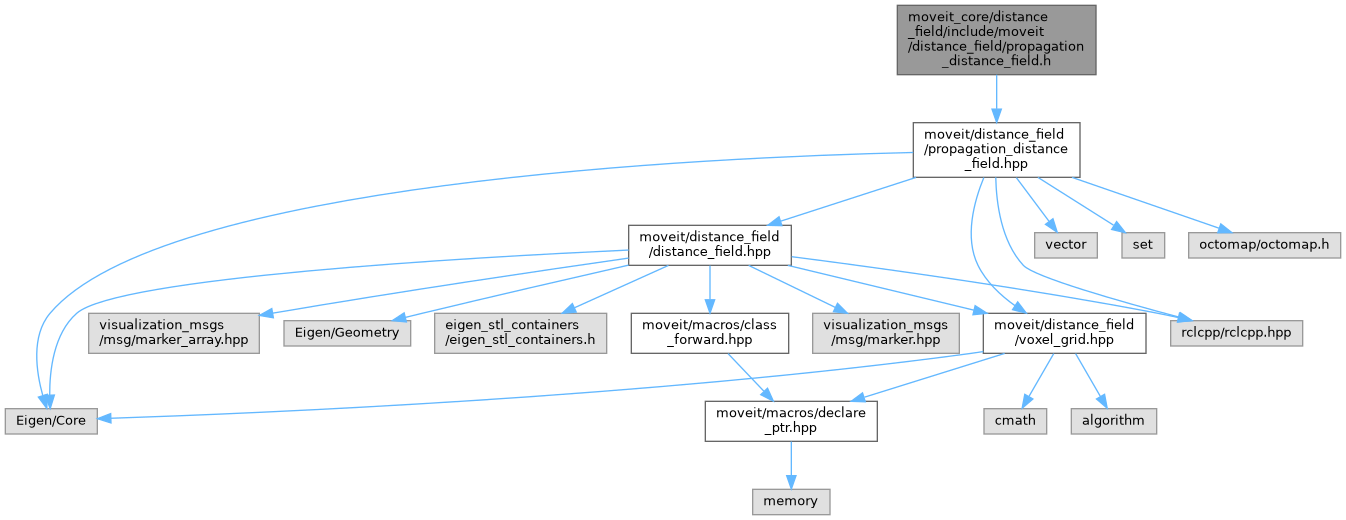moveit2 The MoveIt Motion Planning Framework for ROS 2.
propagation_distance_field.h File Reference
`#include <moveit/distance_field/voxel_grid.h>`
`#include <moveit/distance_field/distance_field.h>`
`#include <vector>`
`#include <Eigen/Core>`
`#include <set>`
`#include <octomap/octomap.h>`
`#include <rclcpp/rclcpp.hpp>`
Include dependency graph for propagation_distance_field.h:This graph shows which files directly or indirectly include this file:Go to the source code of this file.

## Classes

struct  distance_field::CompareEigenVector3i
Struct for sorting type Eigen::Vector3i for use in sorted std containers. Sorts in z order, then y order, then x order. More...

struct  distance_field::PropDistanceFieldVoxel
Structure that holds voxel information for the DistanceField. Will be used in VoxelGrid. More...

class  distance_field::PropagationDistanceField
A DistanceField implementation that uses a vector propagation method. Distances propagate outward from occupied cells, or inwards from unoccupied cells if negative distances are to be computed, which is optional. Outward and inward propagation only occur to a desired maximum distance - cells that are more than this maximum distance from the nearest cell will have maximum distance measurements. More...

## Namespaces

EigenSTL

distance_field
Namespace for holding classes that generate distance fields.

## Typedefs

typedef std::vector< Eigen::Vector3i, Eigen::aligned_allocator< Eigen::Vector3i > > EigenSTL::vector_Vector3i